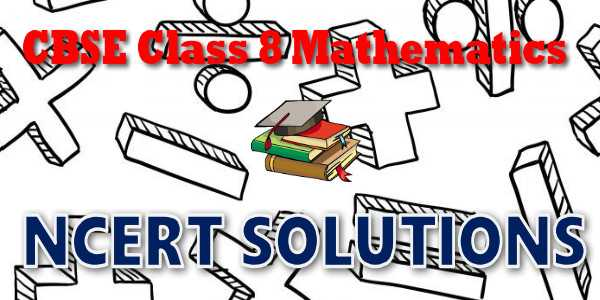No products in the cart.

# CBSE - Class 08 - Mathematics - Direct and Inverse Proportions - NCERT Solutions## CBSE, JEE, NEET, NDA

Question Bank, Mock Tests, Exam Papers

NCERT Solutions, Sample Papers, Notes, Videos

## NCERT Solutions for Mathematics Direct and Inverse Proportions

Free NCERT Solutions Link## myCBSEguide App

Complete Guide for CBSE Students

NCERT Solutions, NCERT Exemplars, Revison Notes, Free Videos, CBSE Papers, MCQ Tests & more.

# NCERT solutions for class 8 Mathematics Direct and Inverse Proportions## NCERT 8 Mathematics Text book Solutions

NCERT 8th class Mathematics book solutions are available in PDF format for free download. These ncert book chapter wise questions and answers are very helpful for CBSE board exam. CBSE recommends NCERT books and most of the questions in CBSE exam are asked from NCERT text books. Class 8 Mathematics chapter wise NCERT solution for Mathematics part 1 and Mathematics part 2 for all the chapters can be downloaded from our website and myCBSEguide mobile app for free.

## NCERT Class 8 Mathematics Chapter-wise Solutions

Maths Part I

• Chapter 1 - Rational Numbers
• Chapter 2 - Linear Equations in One Variable
• Chapter 3 - Understanding Quadrilaterals
• Chapter 4 - Practical Geometry
• Chapter 5 - Data Handling
• Chapter 6 - Squares and Square Roots
• Chapter 7 - Cubes and Cube Roots
• Chapter 8 - Comparing Quantities
• Chapter 9 - Algebraic Expressions and Identities
• Chapter 10 - Visualising Solid Shapes
• Chapter 11 - Mensuration
• Chapter 12 - Exponents and Powers
• Chapter 13 - Direct and Inverse Proportions
• Chapter 14 - Factorisation
• Chapter 15 - Introduction to Graphs
• Chapter 16 - Playing with Numbers

## CBSE class 8th Mathematics have two books. Each book has chapters and topics.

• NCERT Mathematics Book Class 8 Part-1

Here is the list of topics covered under each chapter of class 8 Mathematics NCERT text book.

• Exercise 1.1
• Exercise 1.2

• Exercise 2.1
• Exercise 2.2
• Exercise 2.3
• Exercise 2.4
• Exercise 2.5
• Exercise 2.6

• Exercise 3.1
• Exercise 3.2
• Exercise 3.3
• Exercise 3.4

• Exercise 4.1
• Exercise 4.2
• Exercise 4.3
• Exercise 4.4
• Exercise 4.5

• Exercise 5.1
• Exercise 5.2
• Exercise 5.3

• Exercise 6.1
• Exercise 6.2
• Exercise 6.3
• Exercise 6.4

• Exercise 7.1
• Exercise 7.2

• Exercise 8.1
• Exercise 8.2
• Exercise 8.3

• Exercise 9.1
• Exercise 9.2
• Exercise 9.3
• Exercise 9.4
• Exercise 9.5

### 10. Visualising Solid Shapes

• Exercise 10.1
• Exercise 10.2
• Exercise 10.3

### 11. Mensuration

• Exercise 11.1
• Exercise 11.2
• Exercise 11.3
• Exercise 11.4

### 12. Exponents and Powers

• Exercise 12.1
• Exercise 12.2

### 13. Direct and Inverse Proportions

• Exercise 13.1
• Exercise 13.2

### 14. Factorisation

• Exercise 14.1
• Exercise 14.2
• Exercise 14.3
• Exercise 14.4

### 15. Introduction to Graphs

• Exercise 15.1
• Exercise 15.2
• Exercise 15.3

### 16.Playing with Numbers

• Exercise 16.1
• Exercise 16.2

## NCERT Solutions Chapter 13 Direct and Inverse Proportions

Mohan prepares tea for himself and his sister. He uses 300 mL of water, 2 spoons of sugar, 1 spoon of tea leaves and 50 mL of milk. How much quantity of each item will he need, if he has to make tea for five persons? If two students take 20 minutes to arrange chairs for an assembly, then how much time would five students take to do the same job? We come across many such situations in our day-to-day life, where we need to see variation in one quantity bringing in variation in the other quantity.
For example:
(i) If the number of articles purchased increases, the total cost also increases.
(ii) More the money deposited in a bank, more is the interest earned.
(iii) As the speed of a vehicle increases, the time taken to cover the same distance
decreases.
(iv) For a given job, more the number of workers, less will be the time taken to complete
the work.

## NCERT Solutions for Class 8th Mathematics

NCERT Solutions Class 8 Mathematics PDF (Download) Free from myCBSEguide app and myCBSEguide website. Ncert solution class 8 Mathematics includes text book solutions from  part . NCERT Solutions for CBSE Class 8 Mathematics have total 16 chapters. Class 8 Mathematics ncert Solutions in pdf for free Download are given in this website. Ncert Mathematics class 8 solutions PDF and Mathematics ncert class 8 PDF solutions with latest modifications and as per the latest CBSE syllabus are only available in myCBSEguide.

## NCERT Solutions for Class 5

NCERT Solutions for Class 4

## NCERT Solutions for Class 3## myCBSEguide

Trusted by 1 Crore+ Students

#### Question Paper Creator

• Create papers in minutes
• Print with your name & Logo